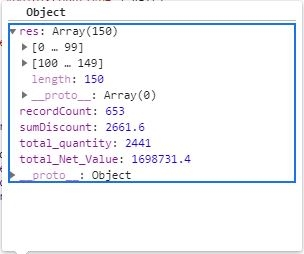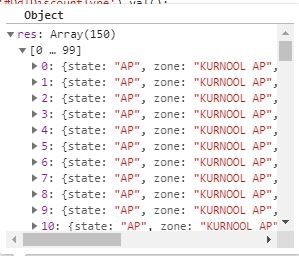# Can we initialize datatable in success property of ajax?

• I copied your code here so we can look at it:

`````` \$('#id_fetch,.filterOkButton').on('click', function () {
debugger;

\$.ajax({
url: '/Reports/_GetDiscountGrid',
data: {
State: stat,
Zone: zon,
Amrac: amr,
Store: store,
Hierarchy: hirarcy,
DiscountType: discountType,
Itemcategorycode: itemcatCode,
Itemcode: code,
Period: period,
OfferNo: offernumber

},
datatype: "html",

success: function (data) {

\$('#tbldiscountDIV').empty();
\$('#tbldiscountDIV').show();
//initializing DataTable

\$('#tblDiscount').DataTable().clear();
\$('#tblDiscount').DataTable().destroy();
var countRecords = data.recordCount;
var totalOfDiscount = data.sumDiscount;
var totalOfNetValue = data.total_Net_Value;
var totalOfQuantity = data.total_quantity;
var dataset = "";
var out = [];
out = data.res;
\$('#tblDiscount').DataTable({
serverSide: true,
ordering: false,
searching: false,
lengthChange: false,
footer: true,
scrollY: 500,
scrollX: true,
scroller: {
},
columnDefs: [{ targets: [14, 16, 17], className: 'dt-body-right' },
{ targets: [0, 1, 2, 3, 4, 5, 6, 7, 8, 9, 10, 11, 12, 13, 15], classname: 'dt-body-left' }
],
dom: 'Bfrtip',
async: false,
ajax: function (data, callback, settings) {
var transferDate = '';
var parsedDate = '';
var expiryDate = '';
var parsedExpiryDate = '';

dataset = data.res;
countRecords = data.recordCount;
totalOfDiscount = data.sumDiscount;
totalOfNetValue = data.total_Net_Value;
totalOfQuantity = data.total_quantity;
\$("#tblDiscount_paginate").hide();

//pushing into array
for (var i = 0; i < data.res.length; i++) {

transferDate = data.res[i].TransDate;
parsedDate = moment(transferDate);
data.res[i].TransDate = parsedDate.format('DD-MM-YYYY');

expiryDate = data.res[i].ExpirationDate;
parsedExpiryDate = moment(expiryDate);
data.res[i].ExpirationDate = parsedExpiryDate.format('DD-MM-YYYY');

if (hirarcy == "I") {
out.push([data.res[i].state, data.res[i].zone,
data.res[i].Depot, data.res[i].StoreCode, data.res[i].OfferType, data.res[i].DiscountType,
data.res[i].OfferNo, data.res[i].Description, data.res[i].ReceiptNo, data.res[i].TransDate,
data.res[i].Category, data.res[i].ItemCode, data.res[i].LotNo, data.res[i].ExpirationDate,
data.res[i].Quantity, data.res[i].FreeItem, data.res[i].NetValue, data.res[i].Discount]);

}
else if (hirarcy == "S") {
out.push([data.res[i].state, data.res[i].zone, data.res[i].amro,
data.res[i].StoreCode, data.res[i].OfferType, data.res[i].DiscountType,
data.res[i].OfferNo, data.res[i].Description, data.res[i].ReceiptNo, data.res[i].TransDate,
data.res[i].Category, data.res[i].ItemCode, data.res[i].LotNo, data.res[i].ExpirationDate,
data.res[i].Quantity, data.res[i].FreeItem, data.res[i].NetValue, data.res[i].Discount]);
}
else if (hirarcy == "F") {
out.push([data.res[i].state, data.res[i].zone, data.res[i].rac,
data.res[i].StoreCode, data.res[i].OfferType, data.res[i].DiscountType,
data.res[i].OfferNo, data.res[i].Description, data.res[i].ReceiptNo, data.res[i].TransDate,
data.res[i].Category, data.res[i].ItemCode, data.res[i].LotNo, data.res[i].ExpirationDate,
data.res[i].Quantity, data.res[i].FreeItem, data.res[i].NetValue, data.res[i].Discount]);
}
};//end of for loop
setTimeout(function () {
callback({
draw: data.draw,
data: out,
recordsTotal: parseInt(countRecords),
recordsFiltered: parseInt(countRecords)
});
}, 150); //scroll request size and limit size is same

}, //end of Datatable ajax
footerCallback: function (row, data, start, end, display) {
var api = this.api()
var json = api.ajax.json();
\$(api.column(17).footer()).html(totalOfDiscount);
var api = this.api(), data;
\$(api.column(17).footer()).html('Total:' + totalOfDiscount);
\$(api.column(16).footer()).html('Total:' + totalOfNetValue);
\$(api.column(14).footer()).html('Total:' + totalOfQuantity);
}

});//end of dataTable

},//end of success for _GetDiscountGrid
error: function(jqXHR, textStatus, errorThrown) {
if (jqXHR.status == 500) {
} else {
'information You are fetching has more than 1 lac Records');
}
}
});

}
);
``````
• Thats a lot of code to look through. It will be hard to debug without actually seeing the page and the data. Can you post a link to your page or a test case replicating the issue?
https://datatables.net/manual/tech-notes/10#How-to-provide-a-test-case

To answer your question - yes you can initialize Datatables from within the `success` function. Not sure I totally get what you are trying to do.

There is no error in console or javascript code. But my dataTable is not drawn.

Does this mean you see an empty Datatable or no Datatable at all?

It seems like what you have is overly complex. You have `serverSide: true,` which is expecting the row data to be paged by the server. Does your server script perform paging or return all of the data?

Can you post a snippet of the JSON response from your server?

Kevin

• Wow, Kevin,, i am not sure what all to post.

But here goes :
1.Does this mean you see an empty Datatable or no Datatable at all?- I am not seeing any dataTable at all.

2.Does your server script perform paging or return all of the data?-- Row data is not paged by server. It just returns the data from Stored Procedure.

•So i am not sure if this would suffice but this is what i am getting JSON response from action controller method.

• Thats a good start, thanks.

Looks like 150 rows of data is return along with some other data points. If you just want to display those 150 rows you could do something as simple as this:
http://live.datatables.net/meqofepo/1/edit

You would use `data.res` instead of `data.data`, for example:

``````    \$.ajax({
url: "/ajax/arrays.txt",
success: function (data) {
data = JSON.parse(data);

\$('#example').DataTable( {
data: data.res
} );
}
});
``````

You are doing some data manipulation in the Datatables `ajax` function. You can move it into the success function before initializing Datatables.

Remove the `serverSide: true` option and the `ajax` option. Lets see if that gets you closer to what you want.

Kevin

• Kevin,
I actually solved this problem and ran into something else now. I achieved the onscroll data load. however, after scroll when the next set of 150 records is supposed to come what is happening that it is showing me previous records. I check edin controllers the data they are sending is correct but why previous set of data is gettig loaded in baffling. Morever ,the new set of data i see for a fraction of second before the grid gets refreshed to previous data automatically.

• Guessing you are calling `draw()` somewhere that is causing it to go to the first page??

Please post a link to your page or a test case replicating the issue so we can take a look.
https://datatables.net/manual/tech-notes/10#How-to-provide-a-test-case

Kevin

• setTimeout(function () {
callback({
draw: data.draw,
data: out,
recordsTotal: parseInt(countRecords),
recordsFiltered: parseInt(countRecords)
});
}, 14);

I think ,this might be the problem.

• ok, let me generate a link so that you can replicate the test. But , how will i replicate the database, i don't know. My data is coming from database.

• You were,right. When i made one of the columns to be displayed in ascending order, i found that after few micro seconds it is going to the first page.

• But i have not yet figured out solution.

It looks like you're fiddling with the data inside `ajax`. Wouldn't it be easier to do that in the `success` function for the ajax call, and then pass that data into DataTables with `data`?

Colin

• Any chance you are talking like this?

``````function initializeDataTable(data) {
\$('#tbldiscountDIV').show();
//function initializeDataTable() {
//\$('#tbldiscountDIV').empty();
//\$('#tbldiscountDIV').show();
\$('#tblDiscount').DataTable().clear();
\$('#tblDiscount').DataTable().destroy();
var dataset = data;
var countRecords = data.recordCount;
var totalOfDiscount = data.sumDiscount;
var totalOfNetValue = data.total_Net_Value;
var totalOfQuantity = data.total_quantity;
var out = [];
var hirarcy = '@Session["HierarchyLevel"]';
\$('#tblDiscount').DataTable({
serverSide: true, // true
ordering: false, // false
searching: false, // false
lengthChange: false, // pagesize

footer: true,
columnDefs: [{ targets: [14, 16, 17], className: 'dt-body-right' },
{ targets: [0, 1, 2, 3, 4, 5, 6, 7, 8, 9, 10, 11, 12, 13, 15], classname: 'dt-body-left' },
{ targets: , orderable: true }
//,{
//    targets: [9,13],
//    render:\$.fn.dataTable.render.moment()
// }
],
//searching: true,
dom: 'frBtip',
async: false,
ajax: function (data, callback, settings) {

var skip = 0;
if (data.start == 0)
skip = 0;
else
skip = data.start + 50; //data.length;

\$.ajax({
url: '../Reports/_GetOnScrollData',
data: {

skipRows: skip,
limit: 50 //data.length
},
datatype: "Json",

async: false,
success: function (dataset) {
//var item = \$.parseJSON(data);
var transferDate = '';
var parsedDate = '';
var expiryDate = '';
var parsedExpiryDate = '';

// dataset = data.res;
countRecords = dataset.recordCount;
totalOfDiscount = dataset.sumDiscount;
totalOfNetValue = dataset.total_Net_Value;
totalOfQuantity = dataset.total_quantity;
\$("#tblDiscount_paginate").hide();

for (var i = 0; i < dataset.res.length; i++) {

transferDate = dataset.res[i].TransDate;
parsedDate = moment(transferDate);
dataset.res[i].TransDate = parsedDate.format('DD-MM-YYYY');

expiryDate = dataset.res[i].ExpirationDate;
parsedExpiryDate = moment(expiryDate);
dataset.res[i].ExpirationDate = parsedExpiryDate.format('DD-MM-YYYY');

if (hirarcy == "I") {
out.push([data.res[i].state, data.res[i].zone,
data.res[i].Depot, data.res[i].StoreCode, data.res[i].OfferType, data.res[i].DiscountType,
data.res[i].OfferNo, data.res[i].Description, data.res[i].ReceiptNo, data.res[i].TransDate,
data.res[i].Category, data.res[i].ItemCode, data.res[i].LotNo, data.res[i].ExpirationDate,
data.res[i].Quantity, data.res[i].FreeItem, data.res[i].NetValue, data.res[i].Discount]);

}
else if (hirarcy == "S") {
out.push([dataset.res[i].state, dataset.res[i].zone, dataset.res[i].amro,
dataset.res[i].StoreCode, dataset.res[i].OfferType, dataset.res[i].DiscountType,
dataset.res[i].OfferNo, dataset.res[i].Description, dataset.res[i].ReceiptNo, dataset.res[i].TransDate,
dataset.res[i].Category, dataset.res[i].ItemCode, dataset.res[i].LotNo, dataset.res[i].ExpirationDate,
dataset.res[i].Quantity, dataset.res[i].FreeItem, dataset.res[i].NetValue, dataset.res[i].Discount]);
}
else if (hirarcy == "F") {
out.push([dataset.res[i].state, dataset.res[i].zone, dataset.res[i].rac,
dataset.res[i].StoreCode, dataset.res[i].OfferType, dataset.res[i].DiscountType,
dataset.res[i].OfferNo, dataset.res[i].Description, dataset.res[i].ReceiptNo, dataset.res[i].TransDate,
dataset.res[i].Category, dataset.res[i].ItemCode, dataset.res[i].LotNo, dataset.res[i].ExpirationDate,
dataset.res[i].Quantity, dataset.res[i].FreeItem, dataset.res[i].NetValue, dataset.res[i].Discount]);
}
}

//console.log(out.length);
},

error: function (jqXHR, textStatus, errorThrown) {
if (jqXHR.status == 500) {
} else {
'information You are fetching has more than 1 lac Records');
}
},

}); //data extraction ajax

setTimeout(function () {
callback({
draw: data.draw,
data: out,
recordsTotal: parseInt(countRecords),
recordsFiltered: parseInt(countRecords)
});
}, 50);
}, //end of ajax call for controller,call back and settings ajax not the data extraction ajax
scrollY: 500, // div height
scrollX: true,
scroller: {
},

footerCallback: function (row, data, start, end, display) {
var api = this.api()
var json = api.ajax.json();
\$(api.column(17).footer()).html(totalOfDiscount);
\$(api.column(16).footer()).html('Total:' + totalOfNetValue);
\$(api.column(14).footer()).html('Total:' + totalOfQuantity);

/*
var api = this.api(), data;

// Remove the formatting to get integer data for summation
var intVal = function (i) {
return typeof i === 'string' ?
i.replace(/[\\$,]/g, '') * 1 :
typeof i === 'number' ?
i : 0;
};

// Total over all pages
total = api
.column(17)
.data()
.reduce(function (a, b) {
return intVal(a) + intVal(b);
}, 0);

pageTotal = api
.column(17, { page: 'current' })
.data()
.reduce(function (a, b) {
return intVal(a) + intVal(b);
}, 0);

//// Update footer
\$(api.column(17).footer()).html('Total:' + totalOfDiscount);

*/
}
}); //end of ajax call for scroller

}

``````
• ``````function initializeDataTable(data) {

\$('#tblDiscount').DataTable().clear();
\$('#tblDiscount').DataTable().destroy();
var dataset = data;
var countRecords = data.recordCount;
var totalOfDiscount = data.sumDiscount;
var totalOfNetValue = data.total_Net_Value;
var totalOfQuantity = data.total_quantity;
var out = [];
var hirarcy = '@Session["HierarchyLevel"]';
\$('#tblDiscount').DataTable({
serverSide: true, // true
ordering: false, // false
searching: false, // false
lengthChange: false, // pagesize

footer: true,
columnDefs: [{ targets: [14, 16, 17], className: 'dt-body-right' },
{ targets: [0, 1, 2, 3, 4, 5, 6, 7, 8, 9, 10, 11, 12, 13, 15], classname: 'dt-body-left' },
{ targets: , orderable: true }
//,{
//    targets: [9,13],
//    render:\$.fn.dataTable.render.moment()
// }
],
//searching: true,
dom: 'frBtip',
async: false,
ajax: function (data, callback, settings) {

var skip = 0;
if (data.start == 0)
skip = 0;
else
skip = data.start + 50; //data.length;

\$.ajax({
url: '../Reports/_GetOnScrollData',
data: {

skipRows: skip,
limit: 50 //data.length
},
datatype: "Json",

async: false,
success: function (dataset) {
//var item = \$.parseJSON(data);
var transferDate = '';
var parsedDate = '';
var expiryDate = '';
var parsedExpiryDate = '';

// dataset = data.res;
countRecords = dataset.recordCount;
totalOfDiscount = dataset.sumDiscount;
totalOfNetValue = dataset.total_Net_Value;
totalOfQuantity = dataset.total_quantity;
\$("#tblDiscount_paginate").hide();

for (var i = 0; i < dataset.res.length; i++) {

transferDate = dataset.res[i].TransDate;
parsedDate = moment(transferDate);
dataset.res[i].TransDate = parsedDate.format('DD-MM-YYYY');

expiryDate = dataset.res[i].ExpirationDate;
parsedExpiryDate = moment(expiryDate);
dataset.res[i].ExpirationDate = parsedExpiryDate.format('DD-MM-YYYY');

if (hirarcy == "I") {
out.push([data.res[i].state, data.res[i].zone,
data.res[i].Depot, data.res[i].StoreCode, data.res[i].OfferType, data.res[i].DiscountType,
data.res[i].OfferNo, data.res[i].Description, data.res[i].ReceiptNo, data.res[i].TransDate,
data.res[i].Category, data.res[i].ItemCode, data.res[i].LotNo, data.res[i].ExpirationDate,
data.res[i].Quantity, data.res[i].FreeItem, data.res[i].NetValue, data.res[i].Discount]);

}
else if (hirarcy == "S") {
out.push([dataset.res[i].state, dataset.res[i].zone, dataset.res[i].amro,
dataset.res[i].StoreCode, dataset.res[i].OfferType, dataset.res[i].DiscountType,
dataset.res[i].OfferNo, dataset.res[i].Description, dataset.res[i].ReceiptNo, dataset.res[i].TransDate,
dataset.res[i].Category, dataset.res[i].ItemCode, dataset.res[i].LotNo, dataset.res[i].ExpirationDate,
dataset.res[i].Quantity, dataset.res[i].FreeItem, dataset.res[i].NetValue, dataset.res[i].Discount]);
}
else if (hirarcy == "F") {
out.push([dataset.res[i].state, dataset.res[i].zone, dataset.res[i].rac,
dataset.res[i].StoreCode, dataset.res[i].OfferType, dataset.res[i].DiscountType,
dataset.res[i].OfferNo, dataset.res[i].Description, dataset.res[i].ReceiptNo, dataset.res[i].TransDate,
dataset.res[i].Category, dataset.res[i].ItemCode, dataset.res[i].LotNo, dataset.res[i].ExpirationDate,
dataset.res[i].Quantity, dataset.res[i].FreeItem, dataset.res[i].NetValue, dataset.res[i].Discount]);
}
}

//console.log(out.length);
},

error: function (jqXHR, textStatus, errorThrown) {
if (jqXHR.status == 500) {
} else {
'information You are fetching has more than 1 lac Records');
}
},

}); //data extraction ajax

setTimeout(function () {
callback({
draw: data.draw,
data: out,
recordsTotal: parseInt(countRecords),
recordsFiltered: parseInt(countRecords)
});
}, 50);
}, //end of ajax call for controller,call back and settings ajax not the data extraction ajax
scrollY: 500, // div height
scrollX: true,
scroller: {
},

footerCallback: function (row, data, start, end, display) {
var api = this.api()
var json = api.ajax.json();
\$(api.column(17).footer()).html(totalOfDiscount);
\$(api.column(16).footer()).html('Total:' + totalOfNetValue);
\$(api.column(14).footer()).html('Total:' + totalOfQuantity);

/*
var api = this.api(), data;

// Remove the formatting to get integer data for summation
var intVal = function (i) {
return typeof i === 'string' ?
i.replace(/[\\$,]/g, '') * 1 :
typeof i === 'number' ?
i : 0;
};

// Total over all pages
total = api
.column(17)
.data()
.reduce(function (a, b) {
return intVal(a) + intVal(b);
}, 0);

pageTotal = api
.column(17, { page: 'current' })
.data()
.reduce(function (a, b) {
return intVal(a) + intVal(b);
}, 0);

//// Update footer
\$(api.column(17).footer()).html('Total:' + totalOfDiscount);

*/
}
}); //end of ajax call for scroller

}

``````
• edited April 8

@colin : are you talking about the above type of code.. it is very complicated what i had to do.